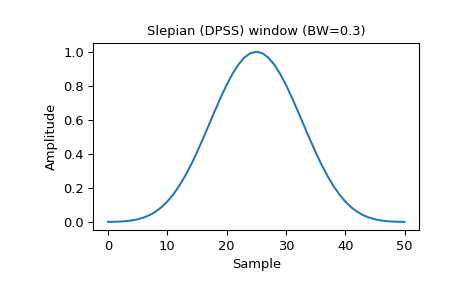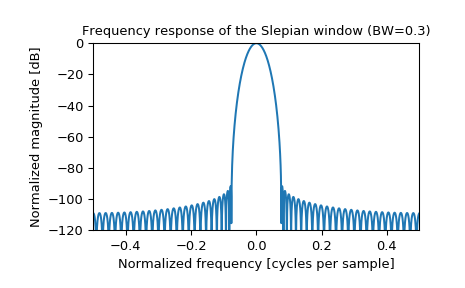#### Previous topic

scipy.signal.parzen

#### Next topic

scipy.signal.triang

# scipy.signal.slepian¶

scipy.signal.slepian(M, width, sym=True)[source]

Return a digital Slepian (DPSS) window.

Used to maximize the energy concentration in the main lobe. Also called the digital prolate spheroidal sequence (DPSS).

Parameters: M : int Number of points in the output window. If zero or less, an empty array is returned. width : float Bandwidth sym : bool, optional When True (default), generates a symmetric window, for use in filter design. When False, generates a periodic window, for use in spectral analysis. w : ndarray The window, with the maximum value always normalized to 1

References

 [R262] D. Slepian & H. O. Pollak: “Prolate spheroidal wave functions, Fourier analysis and uncertainty-I,” Bell Syst. Tech. J., vol.40, pp.43-63, 1961. https://archive.org/details/bstj40-1-43
 [R263] H. J. Landau & H. O. Pollak: “Prolate spheroidal wave functions, Fourier analysis and uncertainty-II,” Bell Syst. Tech. J. , vol.40, pp.65-83, 1961. https://archive.org/details/bstj40-1-65

Examples

Plot the window and its frequency response:

>>> from scipy import signal
>>> from scipy.fftpack import fft, fftshift
>>> import matplotlib.pyplot as plt

>>> window = signal.slepian(51, width=0.3)
>>> plt.plot(window)
>>> plt.title("Slepian (DPSS) window (BW=0.3)")
>>> plt.ylabel("Amplitude")
>>> plt.xlabel("Sample")

>>> plt.figure()
>>> A = fft(window, 2048) / (len(window)/2.0)
>>> freq = np.linspace(-0.5, 0.5, len(A))
>>> response = 20 * np.log10(np.abs(fftshift(A / abs(A).max())))
>>> plt.plot(freq, response)
>>> plt.axis([-0.5, 0.5, -120, 0])
>>> plt.title("Frequency response of the Slepian window (BW=0.3)")
>>> plt.ylabel("Normalized magnitude [dB]")
>>> plt.xlabel("Normalized frequency [cycles per sample]")